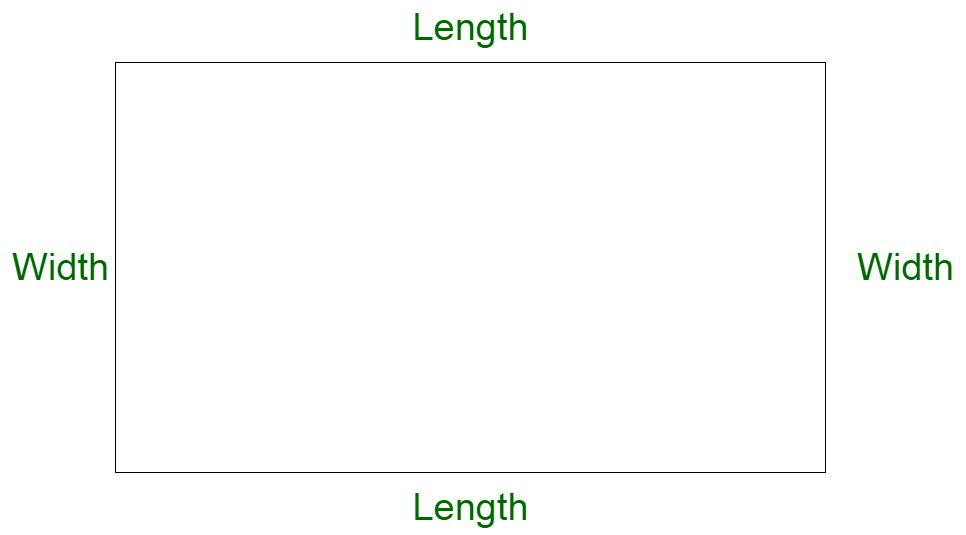Open in App
Not now

# If the length of a rectangle is decreased by 6% and breadth is increased by 6%, calculate the percentage change in the area

• Last Updated : 24 Feb, 2022

Mensuration is a branch of mathematics that deals with geometric shape calculation and its parameters such as area, length, volume, surface area, etc. It deals with both 2D and 3D shapes. Here 2D shapes are those shapes that only have two dimensions such as length and width but no thickness, or have X and Y dimensions. For example, a square, rectangle, triangle, circle, etc. Whereas 3D shapes are those shapes that have 3 dimensions such as length, width, and thickness, or have X, Y, and Z dimensions. For example, bricks, ice-cream cones, football, etc. Mensuration uses mathematical formulas and algebraic equations to solve problems.

Rectangle

A rectangle is a two-dimensional diagram with four sides. The longer side of the rectangle is the length and the shorter side is called width. It is also known as a quadrilateral with four right angles that is 90° each. If all the sides of a rectangle are equal then the shape is known as a square.Rectangle Properties

The properties of a rectangle are given below:

• In a rectangle, the opposite sides are parallel and equal to each other.
• Both the diagonals of the rectangle have the same length.
• Each interior angle of the rectangle is equal to 90 degrees.
• The sum of all the interior angles of the rectangle is equal to 360 degrees.
• Since the sides of a rectangle are parallel, then it is also known as Parallelogram.
• A rectangle is also known as a quadrilateral with four right angles(each of 90°).
• In a rectangle, both the diagonals always bisect each other.

Rectangle Formulae

Area: The area of the rectangle is known as the area occupied by the rectangle within its boundary.

Area of a Rectangle = (Length × Width) square units.

Perimeter: Perimeter of a rectangle is known as the total distance covered by the sides of the rectangle. So we can say that the perimeter of the rectangle is the sum of its four sides.

Perimeter of a Rectangle Formula = 2 * (Length + Width) units.

Diagonal: A diagonal in a rectangle is a line that joins the opposite vertices. a rectangle contains a maximum of two diagonals and both of them are of equal length.

Diagonal of a Rectangle Formula = √Length2 + Width2 units.

### If the length of a rectangle is decreased by 6% and breadth is increased by 6%, calculate the percentage change in the area.

Solution:

Step by step explanation of problem:

Let us considered L, B be length and breadth of rectangle,

then the area of rectangle A1 = L * B.

Here in the question it’s given that the length decreased by 6% and breadth increased by 6%.

so, new length(L1) = L – L * 6%

= L(1 – 6%)

new breadth(B1) = B +  B * 6%

= B(1 + 6%)

new Area of rectangle(A2) = L(1 – 6%) * B(1 + 6%)

= LB(1 – 6% + 6% – (6% * 6%))

= LB(1 – 0.0036)

= 0.9964LB

Now, change in area of rectangles = A2 – A1 = 0.9964LB – LB  = -0.0025LB = -0.36%A1.

Percentage change in area of rectangle = Change in area / Original area

= -0.36%A1 / A1

= -0.36%

Therefore, the percentage change on decreasing the length of a rectangle by 6% and increasing the breadth of a rectangle by 6% is -0.36%. Or we can say that area of rectangle decreases by 0.36% on decreasing the length by 6% and increasing the breadth by 6%.

### Sample Questions

Question 1: The length of a rectangle was increased by 1% and if breadth is decreased by 1% by what percentage did the area of the rectangle change?

Solution:

Percentage Change = l + b + (l * b)/100

l = 1%, b = -1%

Percentage Change = 1 + -1 + (1 x -1)/100 = -0.01

Negative indicates net decrease.

So, the area of the rectangle decreases by 0.01%.

Question 2: The length of a rectangle was increased by 50% and if breadth is decreased by 50% by what percentage did the area of the rectangle change?

Solution:

Percentage Change = l + b + (l * b)/100

l = 50%, b = -50%

Percentage Change = 50 + -50 + (50 x -50)/100 = -25

Negative indicates net decrease.

So, the area of the rectangle decreases by 25%.

Question 3: The length of a rectangle was increased by 10%  by what percentage did the area of the rectangle change?

Solution:

Percentage Change = l + b + (l * b)/100

l = 10%, b = 0%

Percentage Change = 10 + 0 + (10 x 0)/100 = 10

Positive indicates net increase.

So, the area of the rectangle increases by 10%.

My Personal Notes arrow_drop_up